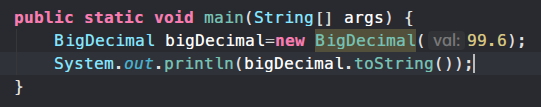﻿ BigDecimal 常用计算方法与注意事项 – 源码巴士

# BigDecimal 常用计算方法与注意事项

`BigDecimal` 是 Java 提供的一个关于小数精确计算的类，其位于`java.math`包下。不同于基本数据类型，`BigDecimal`是调用相关方法来进行运算。因为其拥有非常精确的小数计算能力，所以比较适合用于财务相关的计算等等。但是，其运行效率是不及 `Float``Double` 的，要按照实际情况，运用在合理的地方。

## 对象初始化

``````BigDecimal bigDec = new BigDecimal("99.6");
``````

``````//不建议使用
BigDecimal bigDec = new BigDecimal(99.6);
``````

``````BigDecimal bigDecimal=new BigDecimal("99.6");
System.out.println(bigDecimal.toString());
``````

``````99.6
``````

``````BigDecimal bigDecimal=new BigDecimal(99.6);
System.out.println(bigDecimal.toString());
``````

``````99.599999999999994315658113919198513031005859375
``````## 建议的数据库解决方案

VARCHAR String
DECIMAL BigDecimal

## 加减运算

### 减法

``````BigDecimal bigDecimal = new BigDecimal("99.6");
BigDecimal subtract = bigDecimal.subtract(new BigDecimal("66.9"));
System.out.println(subtract.toString());
System.out.println(bigDecimal.toString());
``````

``````32.7
99.6
``````

``````BigDecimal bigDecimal = new BigDecimal("99.6");
bigDecimal = bigDecimal.subtract(new BigDecimal("66.9"));
System.out.println(bigDecimal.toString());
``````

### 加法

``````BigDecimal bigDecimal = new BigDecimal("99.6");
System.out.println(bigDecimal.toString());
``````

``````166.5
``````

## 乘除运算

### 乘法

``````BigDecimal bigDecimal = new BigDecimal("99.6");
bigDecimal = bigDecimal.multiply(new BigDecimal("66.9"));
System.out.println(bigDecimal.toString());
``````

``````6663.24
``````

### 除法

``````BigDecimal bigDecimal = new BigDecimal("99.6");
bigDecimal = bigDecimal.divide(new BigDecimal("3"));
System.out.println(bigDecimal.toString());
``````

``````33.2
``````

``````BigDecimal bigDecimal = new BigDecimal("99.6");
bigDecimal = bigDecimal.divide(new BigDecimal("66.9"));
System.out.println(bigDecimal.toString());
``````

Exception in thread “main” java.lang.ArithmeticException: Non-terminating decimal expansion; no exact representable decimal result. at java.math.BigDecimal.divide(BigDecimal.java:1690) at …

``````BigDecimal bigDecimal = new BigDecimal("99.6");
bigDecimal = bigDecimal.divide(new BigDecimal("66.9"),2,BigDecimal.ROUND_DOWN);
System.out.println(bigDecimal.toString());
``````

``````1.48
``````

BigDecimal.ROUND_UP 舍入远离零的舍入模式。在丢弃非零部分之前始终增加数字(始终对非零舍弃部分前面的数字加1)。
BigDecimal.ROUND_DOWN 接近零的舍入模式。在丢弃某部分之前始终不增加数字(从不对舍弃部分前面的数字加1，即截短)。
BigDecimal.ROUND_CEILING 接近正无穷大的舍入模式。如果 BigDecimal 为正，则舍入行为与 ROUND_UP 相同；如果为负，则舍入行为与 ROUND_DOWN 相同。
BigDecimal.ROUND_FLOOR 接近负无穷大的舍入模式。如果 BigDecimal 为正，则舍入行为与 ROUND_DOWN 相同；如果为负，则舍入行为与 ROUND_UP 相同。
BigDecimal.ROUND_HALF_UP 向“最接近的”数字舍入，如果与两个相邻数字的距离相等，则为向上舍入的舍入模式。如果舍弃部分 >= 0.5，则舍入行为与 ROUND_UP 相同;否则舍入行为与 ROUND_DOWN 相同。注意，这是我们大多数人在小学时就学过的舍入模式(四舍五入)。
BigDecimal.ROUND_HALF_DOWN 向“最接近的”数字舍入，如果与两个相邻数字的距离相等，则为上舍入的舍入模式。如果舍弃部分 > 0.5，则舍入行为与 ROUND_UP 相同;否则舍入行为与 ROUND_DOWN 相同(五舍六入)。
BigDecimal.ROUND_HALF_EVE 向“最接近的”数字舍入，如果与两个相邻数字的距离相等，则向相邻的偶数舍入。如果舍弃部分左边的数字为奇数，则舍入行为与 ROUND_HALF_UP 相同；如果为偶数，则舍入行为与 ROUND_HALF_DOWN 相同。此舍入模式也称为“银行家舍入法”，主要在美国使用。
BigDecimal.ROUND_UNNECESSARY 断言请求的操作具有精确的结果，因此不需要舍入。如果对获得精确结果的操作指定此舍入模式，则抛出ArithmeticException。

## 比较大小

``````BigDecimal a = new BigDecimal("99.6");
BigDecimal b = new BigDecimal("66.9");
int i = a.compareTo(b);
System.out.println(i);
``````

``````1
``````

`compareTo()`方法的返回值是一个`int`型的数据，以上方示例为例，请看解析

a>b 1
a=b 0
a<b -1

``````BigDecimal a = new BigDecimal("-99.6");
if (a.compareTo(BigDecimal.ZERO) > 0) {
//a是正数
}else if (a.compareTo(BigDecimal.ZERO) == 0){
//a==0
}else {
//a是负数
}
``````

## 其他

### 去除无用的零

``````BigDecimal a = new BigDecimal("99.12300000");
BigDecimal b = a.stripTrailingZeros();
System.out.println(b.toString());
``````

``````99.123
``````